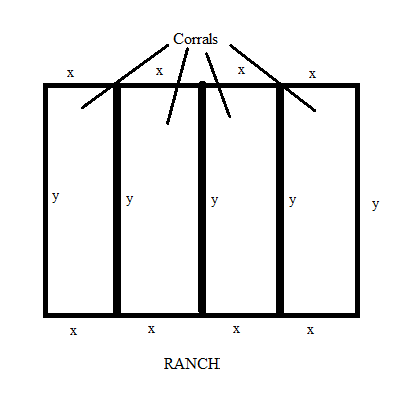# A rancher has 6250 square feet of area which encloses four adjacent rectangular corrals. What is...

## Question:

A rancher has {eq}6250 {/eq} square feet of area which encloses four adjacent rectangular corrals. What is the minimum amount of fencing needed?

## Maxima And Minima Of A Function Using Derivatives :

Given a continuous function {eq}\displaystyle F(x) {/eq}, its absolute extreme (i.e. minima or maxima) can be found by evaluating the function value at its critical points. These critical points can be obtained by equating its first derivative to 0 i.e. {eq}\displaystyle F'(x)=0 {/eq}. The values obtained for {eq}\displaystyle x=x_1,x_2,x_3,\dots {/eq} will give the critical points. At each of these points, there could a potential minima or maxima. This can be checked by calculating the second derivative of the function at these critical points. If {eq}\displaystyle f' '(x)\lt 0 {/eq} at a critical point then the function will be a maxima at that point, but if {eq}\displaystyle f' '(x)\gt 0 {/eq} then the function will have a minima at that point.

Refer the figure shown below• Let each one of the four corrals have a width of {eq}\displaystyle x {/eq} and a height of {eq}\displaystyle y {/eq}.

• So the total perimeter of the rancher will be given by, {eq}\displaystyle \quad \quad (4x+5y+4x)=8x+5y {/eq}.

• Also the rancher area is given to be {eq}\displaystyle 6250 {/eq} sq.ft.

• Now considering the formula for perimeter, {eq}\displaystyle \quad \quad P=8x+5y=8x+5\left( \frac{3125}{2x}\right)=8x+\frac{15625}{2x} {/eq}.

• Differentiating it w.r.t. x and equating to zero. This will give the critical points.

\displaystyle \begin{align} \frac{d}{dx} [P]&=8-\frac{15625}{2x^2}\\ \Rightarrow 0&=8-\frac{15625}{2x^2}\\ \Rightarrow x^2&=\frac{15625}{16}\\ \Rightarrow x&=\sqrt{\frac{15625}{16}}\\ \Rightarrow x&=\frac{125}{4} \end{align}

• Further let us confirm that this value of x will indeed give a minima. For this we find the value of second derivative at {eq}\displaystyle x=\frac{125}{4} {/eq}

$$\displaystyle \quad \quad \frac{d^2}{dx^2}[P]=0+\frac{15625}{x^3} \gt 0$$

• So the second derivative will be +ve for any x value, hence {eq}\displaystyle x=\frac{125}{4} {/eq} will indeed be a minima.

• So the minimum amount of fencing required will be {eq}\displaystyle \quad \quad 8\left( \frac{125}{4}\right)+5(50)=250+250=500 {/eq} feet.